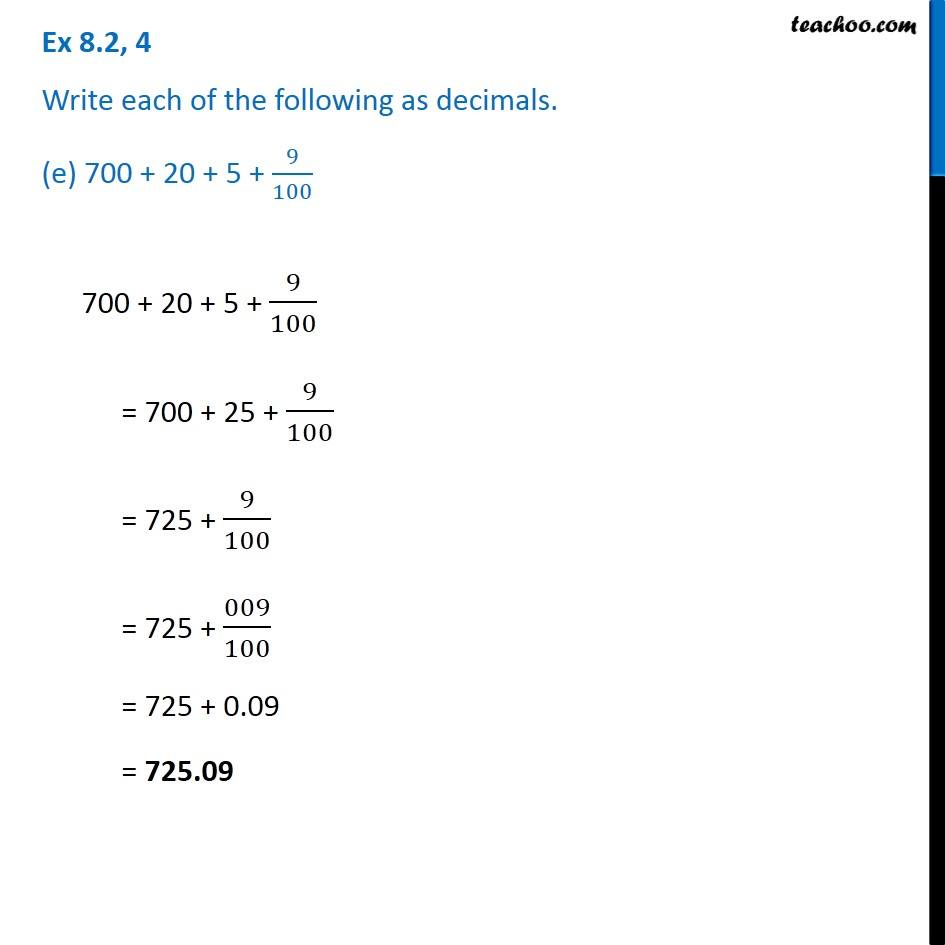Ex 8.2

Chapter 8 Class 6 Decimals
Serial order wiseGet live Maths 1-on-1 Classs - Class 6 to 12

### Transcript

Ex 8.2, 4 Write each of the following as decimals. (e) 700 + 20 + 5 + 9/100 700 + 20 + 5 + 9/100 = 700 + 25 + 9/100 = 725 + 9/100 = 725 + 009/100 = 725 + 0.09 = 725.09